Courses

# Operational Amplifier : Basics - Electronics and Experimental Methods, CSIR-NET Physical Sciences Physics Notes | EduRev

## Physics for IIT JAM, UGC - NET, CSIR NET

Created by: Akhilesh Thakur

## Physics : Operational Amplifier : Basics - Electronics and Experimental Methods, CSIR-NET Physical Sciences Physics Notes | EduRev

The document Operational Amplifier : Basics - Electronics and Experimental Methods, CSIR-NET Physical Sciences Physics Notes | EduRev is a part of the Physics Course Physics for IIT JAM, UGC - NET, CSIR NET.
All you need of Physics at this link: Physics

Operational Amplifiers, or Op-amps as they are more commonly called, are one of the basic building blocks of Analogue Electronic Circuits.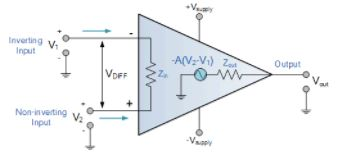Operational amplifiers are linear devices that have all the properties required for nearly ideal DC amplification and are therefore used extensively in signal conditioning, filtering or to perform mathematical operations such as add, subtract, integration and differentiation.

An Operational Amplifier, or op-amp for short, is fundamentally a voltage amplifying device designed to be used with external feedback components such as resistors and capacitors between its output and input terminals. These feedback components determine the resulting function or “operation” of the amplifier and by virtue of the different feedback configurations whether resistive, capacitive or both, the amplifier can perform a variety of different operations, giving rise to its name of “Operational Amplifier”.

An Operational Amplifier is basically a three-terminal device which consists of two high impedance inputs. One of the inputs is called the Inverting Input, marked with a negative or “minus” sign, ( – ). The other input is called the Non-inverting Input, marked with a positive or “plus” sign ( + ).

A third terminal represents the operational amplifiers output port which can both sink and source either a voltage or a current. In a linear operational amplifier, the output signal is the amplification factor, known as the amplifiers gain ( A ) multiplied by the value of the input signal and depending on the nature of these input and output signals, there can be four different classifications of operational amplifier gain.

• Voltage  – Voltage “in” and Voltage “out”

• Current  – Current “in” and Current “out”

• Transconductance  – Voltage “in” and Current “out”

• Transresistance  – Current “in” and Voltage “out”

Since most of the circuits dealing with operational amplifiers are voltage amplifiers, we will limit the tutorials in this section to voltage amplifiers only, (Vin and Vout).

The output voltage signal from an Operational Amplifier is the difference between the signals being applied to its two individual inputs. In other words, an op-amps output signal is the difference between the two input signals as the input stage of an Operational Amplifier is in fact a differential amplifier as shown below.

Differential Amplifier

The circuit below shows a generalized form of a differential amplifier with two inputs marked V1 and V2. The two identical transistors TR1 and TR2 are both biased at the same operating point with their emitters connected together and returned to the common rail, -Vee by way of resistor Re.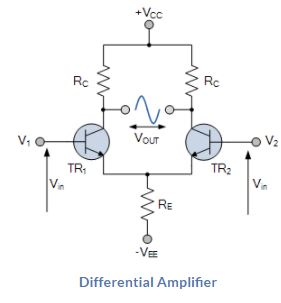The circuit operates from a dual supply +Vcc and -Vee which ensures a constant supply. The voltage that appears at the output, Vout of the amplifier is the difference between the two input signals as the two base inputs are in anti-phasewith each other.

So as the forward bias of transistor, TR1 is increased, the forward bias of transistor TR2 is reduced and vice versa. Then if the two transistors are perfectly matched, the current flowing through the common emitter resistor, Rewill remain constant.

Like the input signal, the output signal is also balanced and since the collector voltages either swing in opposite directions (anti-phase) or in the same direction (in-phase) the output voltage signal, taken from between the two collectors is, assuming a perfectly balanced circuit the zero difference between the two collector voltages.

This is known as the Common Mode of Operation with the common mode gain of the amplifier being the output gain when the input is zero.

Operational Amplifiers also have one output (although there are ones with an additional differential output) of low impedance that is referenced to a common ground terminal and it should ignore any common mode signals that is, if an identical signal is applied to both the inverting and non-inverting inputs there should no change to the output.

However, in real amplifiers there is always some variation and the ratio of the change to the output voltage with regards to the change in the common mode input voltage is called the Common Mode Rejection Ratio or CMRR for short.

Operational Amplifiers on their own have a very high open loop DC gain and by applying some form of Negative Feedback we can produce an operational amplifier circuit that has a very precise gain characteristic that is dependant only on the feedback used. Note that the term “open loop” means that there are no feedback components used around the amplifier so the feedback path or loop is open.

An operational amplifier only responds to the difference between the voltages on its two input terminals, known commonly as the “Differential Input Voltage” and not to their common potential. Then if the same voltage potential is applied to both terminals the resultant output will be zero. An Operational Amplifiers gain is commonly known as the Open Loop Differential Gain, and is given the symbol (Ao).

Equivalent Circuit of an Ideal Operational Amplifier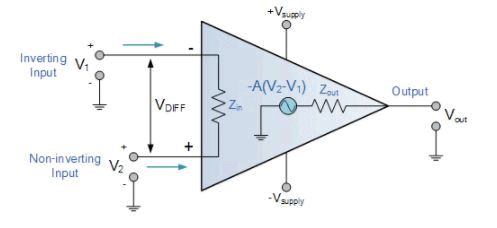Op-amp Parameter and Idealised Characteristic

• Open Loop Gain, (Avo)

• Infinite – The main function of an operational amplifier is to amplify the input signal and the more open loop gain it has the better. Open-loop gain is the gain of the op-amp without positive or negative feedback and for such an amplifier the gain will be infinite but typical real values range from about 20,000 to 200,000.

• Input impedance, (ZIN)

• Infinite – Input impedance is the ratio of input voltage to input current and is assumed to be infinite to prevent any current flowing from the source supply into the amplifiers input circuitry ( IIN = 0 ). Real op-amps have input leakage currents from a few pico-amps to a few milli-amps.

• Output impedance, (ZOUT)

• Zero – The output impedance of the ideal operational amplifier is assumed to be zero acting as a perfect internal voltage source with no internal resistance so that it can supply as much current as necessary to the load. This internal resistance is effectively in series with the load thereby reducing the output voltage available to the load. Real op-amps have output impedances in the 100-20kΩ range.

• Bandwidth, (BW)

• Infinite – An ideal operational amplifier has an infinite frequency response and can amplify any frequency signal from DC to the highest AC frequencies so it is therefore assumed to have an infinite bandwidth. With real op-amps, the bandwidth is limited by the Gain-Bandwidth product (GB), which is equal to the frequency where the amplifiers gain becomes unity.

• Offset Voltage, (VIO)

• Zero – The amplifiers output will be zero when the voltage difference between the inverting and the non-inverting inputs is zero, the same or when both inputs are grounded. Real op-amps have some amount of output offset voltage.

From these “idealized” characteristics above, we can see that the input resistance is infinite, so no current flows into either input terminal (the “current rule”) and that the differential input offset voltage is zero (the “voltage rule”). It is important to remember these two properties as they will help us understand the workings of the Operational Amplifier with regards to the analysis and design of op-amp circuits.

However, real Operational Amplifiers such as the commonly available uA741, for example do not have infinite gain or bandwidth but have a typical “Open Loop Gain” which is defined as the amplifiers output amplification without any external feedback signals connected to it and for a typical operational amplifier is about 100dB at DC (zero Hz). This output gain decreases linearly with frequency down to “Unity Gain” or 1, at about 1MHz and this is shown in the following open loop gain response curve.

Open-loop Frequency Response Curve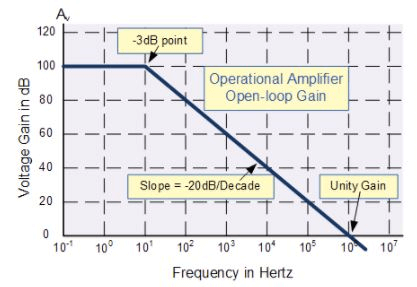From this frequency response curve we can see that the product of the gain against frequency is constant at any point along the curve. Also that the unity gain (0dB) frequency also determines the gain of the amplifier at any point along the curve. This constant is generally known as the Gain Bandwidth Product or GBP. Therefore:

GBP = Gain x Bandwidth = A x BW

For example, from the graph above the gain of the amplifier at 100kHz is given as 20dB or 10, then the gain bandwidth product is calculated as:

GBP = A x BW = 10 x 100,000Hz = 1,000,000.

Similarly, the operational amplifiers gain at 1kHz = 60dB or 1000, therefore the GBP is given as:

GBP = A x BW = 1,000 x 1,000Hz = 1,000,000. The same!.

The Voltage Gain (AV) of the operational amplifier can be found using the following formula: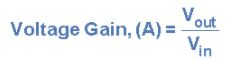and in Decibels or (dB) is given as:An Operational Amplifiers Bandwidth

The operational amplifiers bandwidth is the frequency range over which the voltage gain of the amplifier is above 70.7% or -3dB (where 0dB is the maximum) of its maximum output value as shown below.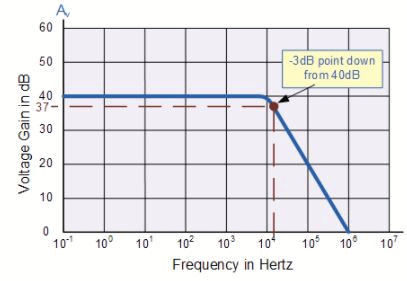Here we have used the 40dB line as an example. The -3dB or 70.7% of Vmax down point from the frequency response curve is given as 37dB. Taking a line across until it intersects with the main GBP curve gives us a frequency point just above the 10kHz line at about 12 to 15kHz. We can now calculate this more accurately as we already know the GBP of the amplifier, in this particular case 1MHz.

Operational Amplifier Example No1.

Using the formula 20 log (A), we can calculate the bandwidth of the amplifier as:

37 = 20 log (A)   therefore, A = anti-log (37 ÷ 20) = 70.8

GBP ÷ A = Bandwidth,  therefore, 1,000,000 ÷ 70.8 = 14,124Hz, or 14kHz

Then the bandwidth of the amplifier at a gain of 40dB is given as 14kHz as previously predicted from the graph.

Operational Amplifier Example No2.

If the gain of the operational amplifier was reduced by half to say 20dB in the above frequency response curve, the -3dB point would now be at 17dB. This would then give the operational amplifier an overall gain of 7.08, therefore A = 7.08.

If we use the same formula as above, this new gain would give us a bandwidth of approximately 141.2kHz, ten times more than the frequency given at the 40dB point. It can therefore be seen that by reducing the overall “open loop gain” of an operational amplifier its bandwidth is increased and visa versa.

In other words, an operational amplifiers bandwidth is inversely proportional to its gain, ( A 1/∞ BW ). Also, this -3dB corner frequency point is generally known as the “half power point”, as the output power of the amplifier is at half its maximum value as shown: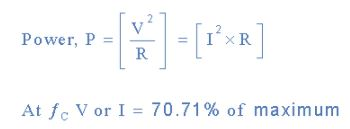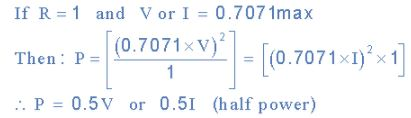Operational Amplifiers Summary

We know now that an Operational amplifiers is a very high gain DC differential amplifier that uses one or more external feedback networks to control its response and characteristics. We can connect external resistors or capacitors to the op-amp in a number of different ways to form basic “building Block” circuits such as, Inverting, Non-Inverting, Voltage Follower, Summing, Differential, Integrator and Differentiator type amplifiers.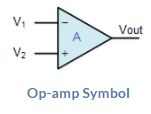Op-amp Symbol

An “ideal” or perfect operational amplifier is a device with certain special characteristics such as infinite open-loop gain AO, infinite input resistance RIN, zero output resistance ROUT, infinite bandwidth 0 to ∞ and zero offset (the output is exactly zero when the input is zero).

There are a very large number of operational amplifier IC’s available to suit every possible application from standard bipolar, precision, high-speed, low-noise, high-voltage, etc, in either standard configuration or with internal Junction FET transistors.

Operational amplifiers are available in IC packages of either single, dual or quad op-amps within one single device. The most commonly available and used of all operational amplifiers in basic electronic kits and projects is the industry standard μA-741.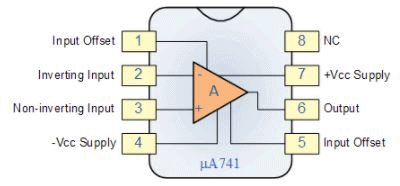159 docs

,

,

,

,

,

,

,

,

,

,

,

,

,

,

,

,

,

,

,

,

,

,

,

,

;